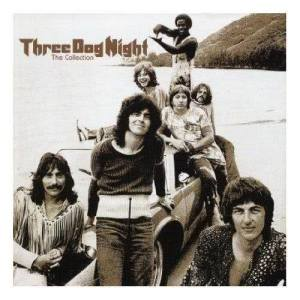# One

Three Dog Night1 Jan 2003

## One Lyrics

One is the loneliest number that you'll ever do

Two can be as bad as one

It's the loneliest number since the number one

No is the saddest experience you'll ever know

Yes it's the saddest experience you'll ever know

'Cause one is the loneliest number that you'll ever do

One is the loneliest number whoa-oh worse than two

It's just no good anymore since you went away

Now I spend my time just making rhymes of yesterday

One is the loneliest number

One is the loneliest number

One is the loneliest number that you'll ever do

One is the loneliest

One is the loneliest

One is the loneliest number that you'll ever do

It's just no good anymore since you went away

Number

One is the loneliest number

One is the loneliest number

One is the loneliest number that you'll ever do

Number

One is the loneliest number

One is the loneliest number

One is the loneliest number that you'll ever do

Number

One one is the loneliest number that you'll ever do

Number

One is the loneliest number that you'll ever do

Number

One is the loneliest number that you'll ever do

***Lyrics are from third-parties***